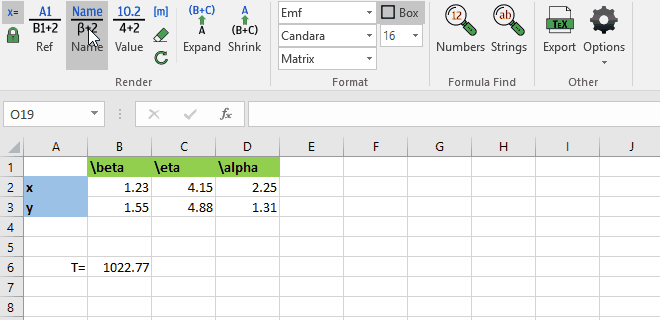# [fx] Render (v5) for Windows Microsoft Excel

#### - revealing the logic within spreadsheet -### Show workings

Turn Excel formulae into graphical equations using associated labels to name variables.

### Check values

Audit and check calculations, by showing the exact values of the variables used in a calculation.

### Dependent Variables

Expand rendered equations to include dependent formulae, showing all variables that influence a calculation.

### Table & Matrices

Summarise matrices or tables using international mathematical notation, with column and row heading recognision.

## Key Features

• Document spreadsheets with illustrated equations of Excel formulas.
• Represent formula variables using cell references, names and label, or values.
• Expand rendered equations to include dependent cells.
• Track equation units.
• Automatic column and row names recognision, and use of named Excel ranges and sheets names.
• Share with anyone, even those without fxRender.
• Customise the equation spacing, style, colour, size, font.
• Use LaTeX mathematical markup to construct intricate labels.
• Export portable PNG or scalable EMF images for use in reports, papers or online.
• Search for constants or text strings hidden within formulas.
• Integrated with the online CodeCogs Equation Editor.